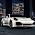# C Programming and Problem Solving Questions and Answers 31 to 40

## C Programming and Problem Solving

### Questions 31 to 40

31.
What is the output of the following code fragment?
void main(){
printf("%x",-1<<4);
}
 (a) fff0 (b) fff1 (c) fff2 (d) fff3 (e) fff4.
32.
Find the output of the following program
void  main(){
char *msg=‘hi’;
printf(msg);
}
 (a) hi (b) h (c) hi followed by garbage charecters (d) Runtime error (e) Garbage output.
31.
Find the output of the following program
void main() {
int i = “hello”;
printf(i);
}
 (a) Syntax error (b) Runtime error (c) Ascii equivalent of character h (d) h (e) hello.
34.
Find the output of the following program
void main() {
int array;
int *i=&array,*j=&array;
int *k=i+j;
int diff=j-i;
printf(“%d”,diff);
}
 (a) Garbage value (b) Runtime error (c) Syntax error (d) 0 (e) 7.
35.
Find the output of the following program
void main()    {
printf(“%d, %d”,sizeof(int *),sizeof(int **));
}
 (a) 2, 0 (b) 0, 2 (c) 0, 0 (d) 2, 2 (e) 1, 1.
36.
What's wrong in this statement?  (x == 4 && y == 5) ? (a = 5) ; (b = 6);
 (a) The question mark should be an equal sign (b) The first semicolon should be a colon (c) There are too many variables in the statement (d) The conditional operator is only used with strings (e) There shouldn’t be any assignment operator in this statement.
37.
Find the output of the following program
void main()      {
int i=10;  /* assume address of i is 0x1234ABCD */
int *ip=&i;
int **ipp=&&i;
printf(“%x,%x,%x”,&i,ip,*ip);
}
 (a) 0x1234ABCD, 0x1234ABCD, 10 (b) 0x1234ABCD, 0x1234ABCD, 0x1234ABCD (c) 0x1234ABCD, 10, 10 (d) Syntax error (e) Runtime error.
38.
Find the output of the following program
void  main() {
int i=01289;
printf(“%d”,i);
}
 (a) 01289 (b) 1289 (c) 713 (d) Syntax error (e) 0713.
39.
Find the output of the following program
void  main() {
int i=065,j=65;
printf(“%d%d”,i,j);
}
 (a) 5365 (b) 6565 (c) 06565 (d) 05365 (e) Syntax error.
40.
Find the value of i that will be printed as the output of the following program
void  main()   {
int i=10;
i=!i>14;
printf ("i=%d",i);
}
 (a) 14 (b) true (c) false (d) 0 (e) 1.

#### Answers

 31. A 1 is Shifted towards left by 4 positions and hence the output is. 32. E The character ‘hi’ is a multi-bye character, and the programmer has typed ‘hi’ instead of “hi” 33. E In the underlying implementation if the size of int and the size of the pointer are same then there is no problem in storing address of the string literal in integer printf is a dump routine and it will interpret the first argument as a string. 34. C We can’t perform addition operation between two pointers. 35. D Any type of pointer is get allocated by 2 bytes of memory in turbo c. 36. B According to the syntax of a ternary operator colon is used along with the ? operator. 37. D && is logical AND operator and this operator requires two operands. 38. D The prefix 0 in an integer value indicates octal value. In octal value use of 8 and 9 is not allowed and hence the error. 39. A As octal 65 ( 065 ) is equivalent of decimal value 53. 40. D In the expression !i>14 , NOT (!) operator has more precedence than ‘ >’ symbol.  ! is a unary logical operator. !i (!10) is 0 (not of true is false).  0>14 is false (zero).

<< PrevNext >>

#### 3 comments :

1.in 32nd i think runtime error would come as 'hi' is considerd as a character to be stored at an index.
https://ideone.com/o4JGzE

2.3.